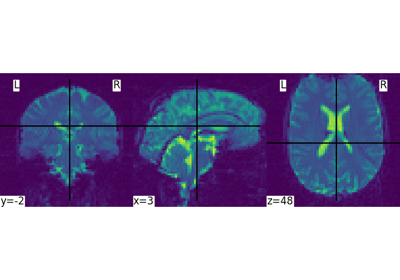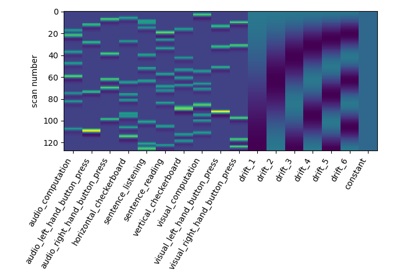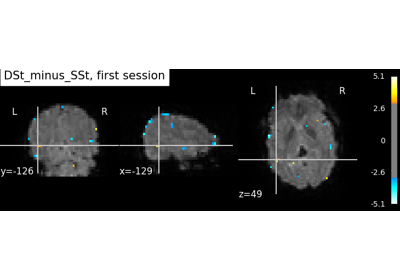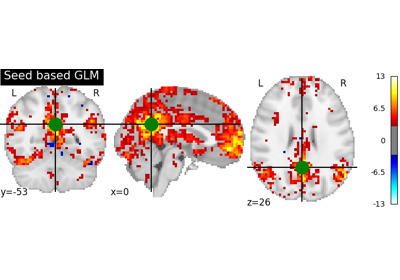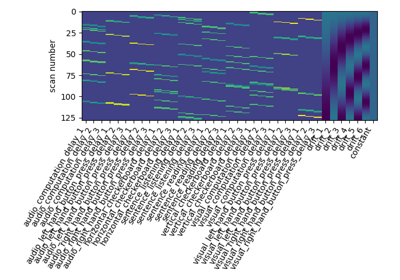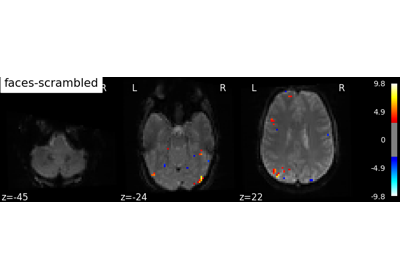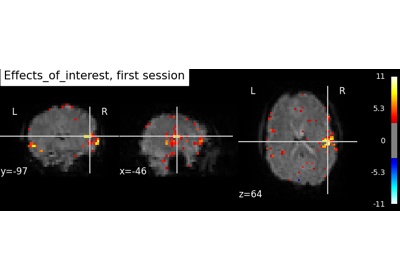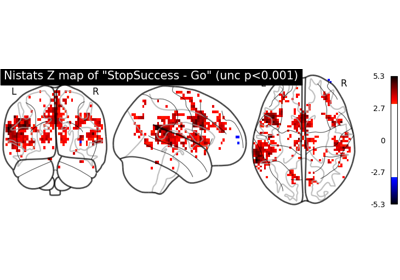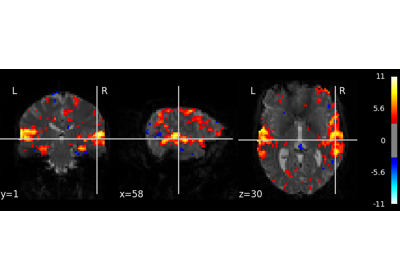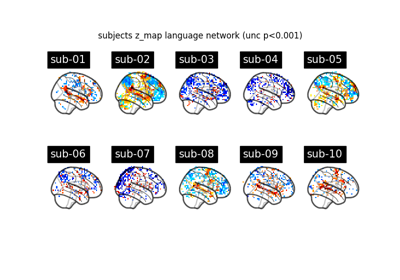Note

This page is a reference documentation. It only explains the class signature, and not how to use it. Please refer to the user guide for the big picture.

3.7.1. nistats.first_level_model.FirstLevelModel¶

class nistats.first_level_model.FirstLevelModel(t_r=None, slice_time_ref=0.0, hrf_model='glover', drift_model='cosine', high_pass=0.01, drift_order=1, fir_delays=, min_onset=-24, mask_img=None, target_affine=None, target_shape=None, smoothing_fwhm=None, memory=Memory(location=None), memory_level=1, standardize=False, signal_scaling=0, noise_model='ar1', verbose=0, n_jobs=1, minimize_memory=True, subject_label=None)

Implementation of the General Linear Model for single session fMRI data.

Parameters
t_rfloat

This parameter indicates repetition times of the experimental runs. In seconds. It is necessary to correctly consider times in the design matrix. This parameter is also passed to nilearn.signal.clean. Please see the related documentation for details.

slice_time_reffloat, optional (default 0.)

This parameter indicates the time of the reference slice used in the slice timing preprocessing step of the experimental runs. It is expressed as a percentage of the t_r (time repetition), so it can have values between 0. and 1.

hrf_model{‘spm’, ‘spm + derivative’, ‘spm + derivative + dispersion’,

‘glover’, ‘glover + derivative’, ‘glover + derivative + dispersion’, ‘fir’, None} String that specifies the hemodynamic response function. Defaults to ‘glover’.

drift_modelstring, optional

This parameter specifies the desired drift model for the design matrices. It can be ‘polynomial’, ‘cosine’ or None.

high_passfloat, optional

This parameter specifies the cut frequency of the high-pass filter in Hz for the design matrices. Used only if drift_model is ‘cosine’.

drift_orderint, optional

This parameter specifices the order of the drift model (in case it is polynomial) for the design matrices.

fir_delaysarray of shape(n_onsets) or list, optional

In case of FIR design, yields the array of delays used in the FIR model, in scans.

min_onsetfloat, optional

This parameter specifies the minimal onset relative to the design (in seconds). Events that start before (slice_time_ref * t_r + min_onset) are not considered.

Mask to be used on data. If an instance of masker is passed, then its mask will be used. If no mask is given, it will be computed automatically by a NiftiMasker with default parameters. If False is given then the data will not be masked.

target_affine3x3 or 4x4 matrix, optional

This parameter is passed to nilearn.image.resample_img. Please see the related documentation for details.

target_shape3-tuple of integers, optional

This parameter is passed to nilearn.image.resample_img. Please see the related documentation for details.

smoothing_fwhmfloat, optional

If smoothing_fwhm is not None, it gives the size in millimeters of the spatial smoothing to apply to the signal.

memorystring, optional

Path to the directory used to cache the masking process and the glm fit. By default, no caching is done. Creates instance of joblib.Memory.

memory_levelinteger, optional

Rough estimator of the amount of memory used by caching. Higher value means more memory for caching.

standardizeboolean, optional

If standardize is True, the time-series are centered and normed: their variance is put to 1 in the time dimension.

signal_scalingFalse, int or (int, int), optional,

If not False, fMRI signals are scaled to the mean value of scaling_axis given, which can be 0, 1 or (0, 1). 0 refers to mean scaling each voxel with respect to time, 1 refers to mean scaling each time point with respect to all voxels & (0, 1) refers to scaling with respect to voxels and time, which is known as grand mean scaling. Incompatible with standardize (standardize=False is enforced when signal_scaling is not False).

noise_model{‘ar1’, ‘ols’}, optional

The temporal variance model. Defaults to ‘ar1’

verboseinteger, optional

Indicate the level of verbosity. By default, nothing is printed. If 0 prints nothing. If 1 prints progress by computation of each run. If 2 prints timing details of masker and GLM. If 3 prints masker computation details.

n_jobsinteger, optional

The number of CPUs to use to do the computation. -1 means ‘all CPUs’, -2 ‘all CPUs but one’, and so on.

minimize_memoryboolean, optional

Gets rid of some variables on the model fit results that are not necessary for contrast computation and would only be useful for further inspection of model details. This has an important impact on memory consumption. True by default.

subject_labelstring, optional

This id will be used to identify a FirstLevelModel when passed to a SecondLevelModel object.

Attributes
labels_array of shape (n_voxels,),

a map of values on voxels used to identify the corresponding model

results_dict,

with keys corresponding to the different labels values. Values are SimpleRegressionResults corresponding to the voxels, if minimize_memory is True, RegressionResults if minimize_memory is False

__init__(self, t_r=None, slice_time_ref=0.0, hrf_model='glover', drift_model='cosine', high_pass=0.01, drift_order=1, fir_delays=, min_onset=-24, mask_img=None, target_affine=None, target_shape=None, smoothing_fwhm=None, memory=Memory(location=None), memory_level=1, standardize=False, signal_scaling=0, noise_model='ar1', verbose=0, n_jobs=1, minimize_memory=True, subject_label=None)

Initialize self. See help(type(self)) for accurate signature.

compute_contrast(self, contrast_def, stat_type=None, output_type='z_score')

Generate different outputs corresponding to the contrasts provided e.g. z_map, t_map, effects and variance. In multi-session case, outputs the fixed effects map.

Parameters
contrast_defstr or array of shape (n_col) or list of (string or

array of shape (n_col))

where n_col is the number of columns of the design matrix, (one array per run). If only one array is provided when there are several runs, it will be assumed that the same contrast is desired for all runs. The string can be a formula compatible with pandas.DataFrame.eval. Basically one can use the name of the conditions as they appear in the design matrix of the fitted model combined with operators +- and combined with numbers with operators +-*/.

stat_type{‘t’, ‘F’}, optional

type of the contrast

output_typestr, optional

Type of the output map. Can be ‘z_score’, ‘stat’, ‘p_value’, ‘effect_size’, ‘effect_variance’ or ‘all’

Returns
outputNifti1Image or dict

The desired output image(s). If output_type == 'all', then the output is a dictionary of images, keyed by the type of image.

fit(self, run_imgs, events=None, confounds=None, design_matrices=None)

Fit the GLM

For each run: 1. create design matrix X 2. do a masker job: fMRI_data -> Y 3. fit regression to (Y, X)

Parameters
run_imgs: Niimg-like object or list of Niimg-like objects,

See http://nilearn.github.io/manipulating_images/input_output.html#inputing-data-file-names-or-image-objects # noqa:E501 Data on which the GLM will be fitted. If this is a list, the affine is considered the same for all.

events: pandas Dataframe or string or list of pandas DataFrames or

strings

fMRI events used to build design matrices. One events object expected per run_img. Ignored in case designs is not None. If string, then a path to a csv file is expected.

confounds: pandas Dataframe or string or list of pandas DataFrames or

strings

Each column in a DataFrame corresponds to a confound variable to be included in the regression model of the respective run_img. The number of rows must match the number of volumes in the respective run_img. Ignored in case designs is not None. If string, then a path to a csv file is expected.

design_matrices: pandas DataFrame or list of pandas DataFrames,

Design matrices that will be used to fit the GLM. If given it takes precedence over events and confounds.

fit_transform(self, X, y=None, **fit_params)

Fit to data, then transform it.

Fits transformer to X and y with optional parameters fit_params and returns a transformed version of X.

Parameters
Xnumpy array of shape [n_samples, n_features]

Training set.

ynumpy array of shape [n_samples]

Target values.

**fit_paramsdict

Returns
X_newnumpy array of shape [n_samples, n_features_new]

Transformed array.

get_params(self, deep=True)

Get parameters for this estimator.

Parameters
deepbool, default=True

If True, will return the parameters for this estimator and contained subobjects that are estimators.

Returns
paramsmapping of string to any

Parameter names mapped to their values.

predicted(self)

Transform voxelwise predicted values to the same shape as the input Nifti1Image(s)

Returns
outputlist

a list of Nifti1Image(s)

r_square(self)

Transform voxelwise r-squared values to the same shape as the input Nifti1Image(s)

Returns
outputlist

a list of Nifti1Image(s)

residuals(self)

Transform voxelwise residuals to the same shape as the input Nifti1Image(s)

Returns
outputlist

a list of Nifti1Image(s)

set_params(self, **params)

Set the parameters of this estimator.

The method works on simple estimators as well as on nested objects (such as pipelines). The latter have parameters of the form <component>__<parameter> so that it’s possible to update each component of a nested object.

Parameters
**paramsdict

Estimator parameters.

Returns
selfobject

Estimator instance.

3.7.1.1. Examples using nistats.first_level_model.FirstLevelModel¶